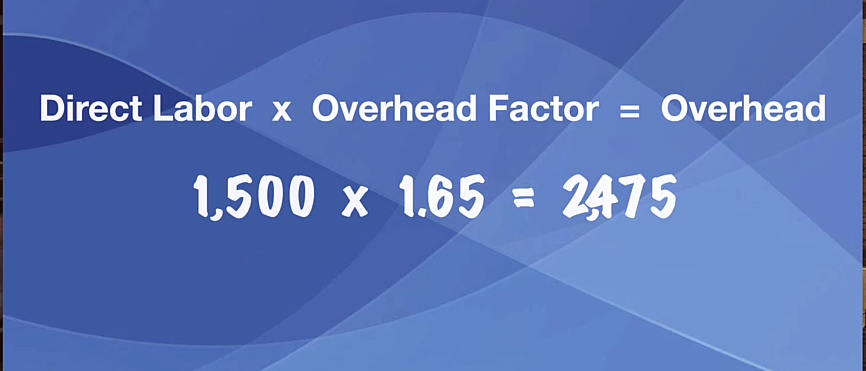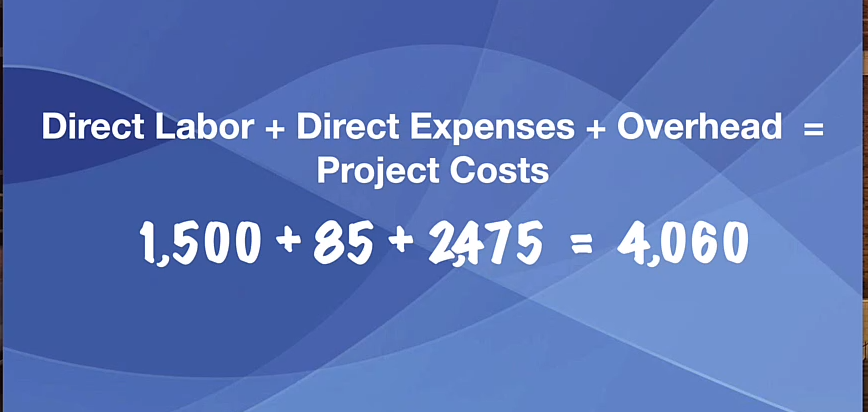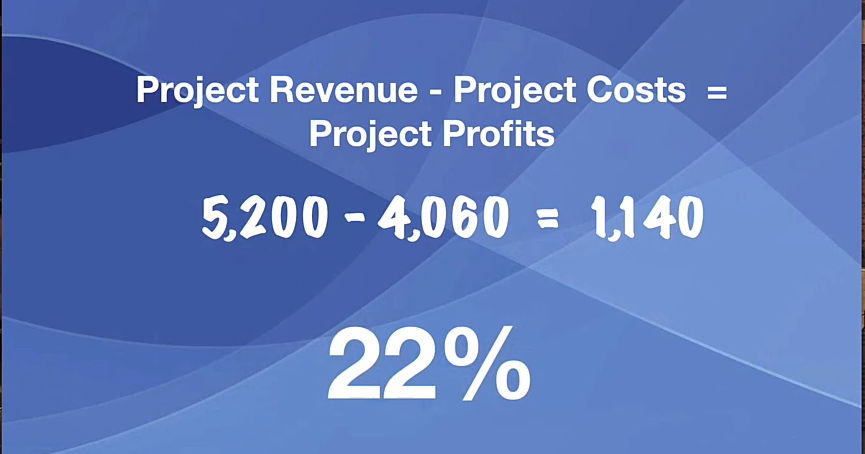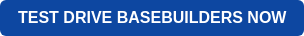In a separate post, we talked about how you’re going to calculate your overhead factor. You can check it out first before you dive into this post.In the United States, the average overhead factor is 1.65, which means that for every dollar of direct labor you have \$1.65 of overhead. We have clients that go as low as \$1, and there are those that go as high as \$2. Your firm's overhead factor should fall somewhere in that range, and the best way to know where your firm stands is to learn how to calculate your overhead factor.

## Put it to Work

Take your direct labor for a given project, multiply it times the overhead factor, and that will give you the overhead that needs to be burdened to that project. So if we had \$1500 of direct labor, we multiply times 1.65, which is the average overhead factor in the United States, and that would give us \$2,475 of overhead.## Project Cost

To calculate the total project cost, we're going to have our direct labor plus any direct expenses, plus overhead gives you your project cost. In this example, we're going to take the \$1,500 and then we’re going to assume \$85 of direct expenses. Then you add the \$2,475. We end up with \$4,060 of total project cost.## Project Profits

Now we can calculate the project profits. We're simply going to take the project revenue, subtract the project cost, and that will give us the project profits.

Taking the \$5,200 minus the \$4,060 gives us \$1,140 of profits, and that's a whopping 22%. Having project profits north of 20% is a great goal to hit.